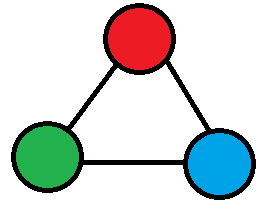# Algorithms: Balancing

Monday, Feb 6, 2017
Section: Home / Post
Categories: Computer Science,
Tags: Algorithms, Graphs,

Balancing in algorithms refers to minimizing the complexity of an algorithm by making sure that its constituent parts share the load efficiently. It is not a technique for solving problems. Instead it helps us understand how an existing solution may be optimized.

## The theory of balancing

Say there is a problem of size $$n$$. The problem is such that it can be broken down into a sequence of smaller problems. There are many ways the problem can be broken down:

Solve the problem in 1 chunk: $$T(n) = f(n)$$

Or solve the problem in chunks of 5: $$T(n) = f(5) + f(n/5)$$

Of course, the relations above are not unique. There are a multitude of ways that problems can be abstracted. But the question arises: what division of load is best?

Let’s assume we want to break down the problem into $$g(n)$$ chunks. Then the size of the sub-problem becomes $$n/g(n)$$. The time to solve the problem becomes: $$T(n) = f(g(n)) + f(n/g(n))$$

In $$big-O$$ notation: $$O(T(n)) = O(f(g(n))) + O(f(n/g(n)))$$

We need to minimize $$O(T(n))$$. Notice that the sum will be dominated by whichever term is larger on the right hand side. Which means that $$f(g(n))$$ and $$f(n/g(n))$$ must be within a constant factor of each other. Essentially, in $$big-O$$ terms: $$f(g(n)) = f(n/g(n))$$

Solving this for $$g(n)$$ gives us the ideal size for partitioning the problem. For simplicity, assume that $$f(n) = n$$, then: $$g(n) = {n \over g(n)}$$ $$\therefore g(n) = \sqrt{n}$$

## Example: The egg dropping problem

Problem: Say you have 2 eggs and a building with $$n$$ storeys. You want to find the storey that will cause the egg to break when dropped from it. What is the fastest way to figure it out?

Solution: A trivial approach is to drop one egg from storeys 1 to $$n$$ until it breaks. This is going to take $$n = O(n)$$ attempts. Good, but we can do better.

There are two eggs. Let’s drop one egg every 5 storeys. Then if the egg breaks on the $$k^{th}$$ attempt we will know that the ‘fatal’ storey is between $$(k-1)\times 5$$ and $$k \times 5$$ storeys. Then the second egg will be dropped from the 5 storeys from $$(k-1)\times 5$$ to $$k \times 5 - 1$$. Therefore the total attempts would be: $${n \over 5} + 5$$

Which is less than $$n$$ but in $$big-O$$ notation has the same complexity: $$O({n \over 5} + 5) = O(n)$$

Our approach with using two eggs is sound. It is reducing total attempts. Let’s generalize the solution. Say the egg is dropped every $$g(n)$$ storeys for a total of $$n/g(n)$$ attempts. Then, like before, the second egg will only be dropped $$g(n)$$ times. This gives the total attempts as: $${n \over g(n)} + g(n)$$

To minimize the total complexity (attempts) the two stages of the solution need to be equally partitioned so one stage does not dominate the other. $$\therefore {n \over g(n)} = g(n)$$ $$g(n) = \sqrt{n}$$

Thus if the first egg makes $$\sqrt{n}$$ drops over the same interval, then the second egg will have to make only $$\sqrt{n}$$ drops, giving the total of: $$\sqrt{n} + \sqrt{n} = O(\sqrt{n}) \lt O(n)$$

## Example: Graph colouring

Problem: Colour a 3-Colourable graph in polynomial time with as few colours as possible.

Solution: A graph is said to be $$n$$-colourable if all vertices can be assigned 1 of $$n$$ colours without adjacent vertices having the same colour. Graph colouring is an NP-Complete problem (except for 1 and 2 colouring). This means that an optimal solution cannot be found in polynomial time. Colouring a 3-colourable graph with exactly 3 colours might be hard, but we can attempt to use as few colours as possible in polynomial time.

One approach is called Greedy colouring. We look at all vertices in a sequence. Each vertex is assigned the first “available” colour. A colour is “available” if it is not assigned to any of the vertex’s neighbours. So if a graph has a maximum degree $$d$$, then the worst case scenario for greedy colouring will take $$d+1$$ colours.

The greedy approach, however, is not leveraging what we know about our graph: it is 3-colourable. Which means that every vertex’s neighbourhood is 2-colourable! 2-colouring is a simple problem. Essentially do any traversal of a graph and switch between 2 colours for each new vertex. We can use this to convert our problem into a sequence of 2- and greedy- colourings.A triangle is the simplest 3-colourable graph. Each vertex's neighbourhood is 2-colourable.

Here is how the new solution works: consider all vertices of degree $$\gt g(n)$$. For each such vertex, 2-colour its neighbourhood. Never use those colours again. Delete the neighbourhood from the graph. Greedily colour the remaining graph. The 2-colouring step will happen at most $$n/g(n)$$ times (since we remove at least $$g(n)$$ vertices each step). So it will use $$O(n/g(n))$$ colours. After the 2-colouring step, only vertices with degree < $$g(n)$$ will remain, which will take $$O(g(n))$$ colours. So the total number of colours will be: $$O({n \over g(n)}) + O(g(n))$$

Balancing both stages gives us: $${n \over g(n)} = g(n) \Rightarrow g(n) = \sqrt{n}$$

Therefore, 2-colouring all vertices with degree $$\gt \sqrt{n}$$ and greedy colouring the remaining vertices will take $$O(\sqrt{n})$$ colours. This is called Widgerson’s Algorithm after (surprise!) Avi Widgerson.

Balancing may not apply to all approaches. Nonetheless it is a powerful tool for analysis of algorithms.